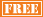January 26
March 9
May 4
June 1

February 9
April 13
June 8

April 20

• ## 2013 GED Test Dates

Varies by State

• ## 2013 AP Exams Test Dates

Calculus AB May 8
Calculus BC May 8
Statistics May 10
Macroeconomics May 16
Microeconomics May 16

# GED Math

We provide intensive tutoring for students preparing for the mathematics section of the GED exam. The mathematics section focuses on 4 general topics; Number operations/number sense, measurement and geometry, data analysis/probability/statistics, and algebra/functions and patterns. There are 2 parts to the mathematics section, each 25 questions with a total of 90 minutes provided for completing the questions. Students must receive a minimum score of 410 on the mathematics section and an overall GED composite score of 450 to pass the exam.

We utilize a two phase attack to help students properly prepare for the mathematics section of the GED Exam. During the course of tutoring sessions, we review numerous simulated GED problems, covering the necessary topics for which students are responsible. Secondly, we reinforce GED syllabus math topics which arise during the review of simulated problems for which students are not sufficiently knowledgeable. These 2 components of our GED prep program provide students with exam problem recognition the day of the exam and the necessary underlying math foundation to solve problems correctly.

We offer a dynamic, intensive program for students preparing for the mathematics section of the GED Exam. Our concentration and expertise is the math section. All of our efforts are directed at the one goal of helping students achieve their highest possible score on the mathematics section of the GED Exam.

• ## One-on-One GED Math Program includes:

Initial student consultationInitial student assessmentNumber of sessions determined by student

Review of simulated GED math problems

Review of strategies for attacking GED math problems

Review of GED math syllabus

Homework review following each session

• ## Group GED Math Program includes:

Initial student consultationInitial student assessmentClass size of no more than 20 students

Review of simulated GED math problems

Review of strategies for attacking GED math problems

Review of GED math syllabus